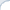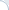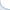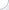If you are programming in C/C++, you can use Single-Instruction Multiple Data(SIMD) commands. The beauty of these commands is that they operate on small vectors rather than singular scalar values. For example, we can multiply or subtract 4 pairs of floating point numbers at the same time. SIMD commands are available on many CPUs, but my post is Intel-specific.

The current standard set of instructions on Intel SSE 4 supports vector operations on 128-bit data elements. The vector size in bits is always 128, but the size of data elements is different. You can operate on sixteen 8-bit values, eight 16-bit values, four 32-bit values, and two 64-bit values. The type of data elements can also be different. A 32-bit value, e.g., can be an integer or a single-precision floating point number. A 64-bit value can be an integer, or a double-precision floating value. Longer, e.g., 256-bit vectors are also supported by newer CPUs, but I do not consider them here.

And it is kinda nightmarish, because you cannot use your regular '+', '-', '*' any more. You have a separate function for each data type (and, even worse, a bunch of conversion functions). For instance, addition of two 4-element floating point vectors is _mm_add_ps, addition of two 2-element double-precision floating point vectors is _mm_add_pd, and addition of two 4-element integer vectors is _mm_add_epi32

It is bad, but not terribly bad, because there is a naming convention that helps you navigate through this jungle. As you might have noticed, all operations start with the same prefix _mm_, then there is a part indicating the type of the operation, and, finally, a type-specific suffix. These suffixes are as follows:

epi8 for 8-bit integers;
epi16 for 16-bit integers;
epi32 for 32-bit integers;
ps for single-precision floating point numbers;
pd for double-precision floating point numbers.

To operate on 128-bit vectors, the CPU uses special 128-bit registers. If you need to extract specific vector elements and store them in regular 32-bit or 64-bit registers, you have to use a special CPU command. Of course, you can always copy vector values to the memory and read back only a necessary portion, but this is rather slow. This is why there are commands that can copy specific elements of a 128-bit vector to a 32-bit or 64-bit CPU register. BTW, store and load operations also follow a convention. The store command for four-element single-precision floating point vectors is _mm_storeu_ps (u in storeu denotes an unaligned write).

The command _mm_exctract_epi8 treats a 128-bit register as a 16-element integer vector. It allows one to extract any of the sixteen integer vector elements (each has a size of 8 bit). _mm_extract_epi16 gives you one of the eight 16-bit vector elements_mm_extract_epi32 extracts one of the four 32-bit integer values. Ok, what does _mm_extract_ps do? Extracts one of the four single-precision floating point numbers, right? Wrong, it also extracts one of the four 32-bit integers. Furthermore, there is no function _mm_extract_pd!

To efficiently extract floating point numbers you need to use functions _mm_cvtss_f32 and _mm_cvtsd_f64. They extract only the first floating point number from the vector. Yet, there is a command to move an arbitrary element of the four-element vector to the first position. This command is called a shuffle instruction. Thus, you can first shuffle an arbitrary element to the first position, and then extract the first element. The name of the shuffle command is a bit of misnomer itself, because shuffle usually means rearranging. Yet, shuffling on Intel is IMHO multiplexing.

It does not bother me much that the actual floating-point extraction functions are missing. Yet, I cannot understand why there is a function _mm_extract_ps with a misleading name and redundant functionality? Anyway, after reading some material on the Web, I have created two simple macros: one for extraction of single-precision and and another for extraction of double-precision floating point numbers. My code is freely available.